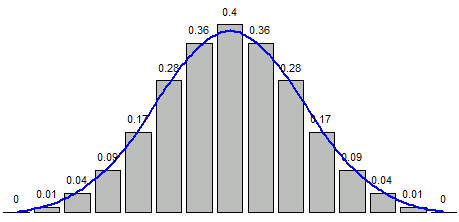# ML Wiki

## Simulation in R

### Distributions

Name RNG PDF CDF
Beta Distribution rbeta dbeta pbeta
Binomial Distribution rbinom dbinom pbinom
Cauchy Distribution rcauchy dcauchy pcauchy
$\chi^2$ Distribution rchisq dchisq pchisq
Exponential Distribution rexp dexp pexp
F Distribution rf df pf
Gamma Distribution rgamma dgamma pgamma
Geometric Distribution rgeom dgeom pgeom
Hypergeometric Distribution rhyper dhyper phyper
Logistic Distribution rlogis dlogis plogis
Log Normal Distribution rlnorm dlnorm plnorm
Negative Binomial Distribution rnbinom dnbinom pnbinom
Normal Distribution rnorm dnorm pnorm
Poisson Distribution rpois dpois ppois
$t$ Distribution rt dt pt
Uniform Distribution runif dunif punif
Weibull Distribution rweibull dweibull pweibull

### rname: Random Number Generator

Example 1

heights = rnorm(10, mean=188, sd=3)
> 186.0 191.2 187.6 187.9 186.6 187.2 187.2 189.5 190.8 186.4


Example 2

• Generates 10 random values from Binomial Distribution
• flipping a coin 10 times = 10 independent experiments with probability 0.5
coinFlips = rbinom(10, size=10, prob=0.5)
> 3 4 6 5 7 6 5 8 5 6


### dname: Probability Density Function

Calculates the density of some probability distribution

x = seq(from=-5, to=5, length=10)
normalDensity = dnorm(x, mean=0, sd=1)
round(normalDensity, 2)
 0.00 0.00 0.01 0.10 0.34 0.34 0.10 0.01 0.00 0.00


Same with 15 :

x = seq(from=-3, to=3, length=15)
normalDensity = dnorm(x, mean=0, sd=1)
r = round(normalDensity, 2)
bp = barplot(r)
xspline(x=bp, y=r, lwd=2, shape=1, border="blue")
text(x=bp, y=r+0.03, labels=as.character(r), xpd=TRUE, cex=0.7)


Code  

So we can see that it generates the values of the density function

•• may be useful for Statistical Tests of Significance

Same for the Binomial distribution:

x = seq(0, 10, by=1)
binomialDensity = dbinom(x, size=10, prob=0.5)
round(binomialDensity,2)


### pname: Cumulative Distribution Function

When you need to know what is the probability of $X \geqslant x$ for some $x$.

For example, you're doing an $F$-Test

• you obtained $F = 3.446$
• $F$ statistic follows the $F$ Distribution: $F \sim F(\text{df1}, \text{df2})$
• so you can calculate the $p$-value:
1 - pf(3.446, df1=1, df2=85)


## Sampling

Function sample draws a random sample

• function(x, size, replace=FALSE, prob=NULL)
• replace=T for sampling with replacement
s = seq(0, 20)
> 0  1  2  3  4  5  6  7  8  9 10 11 12 13 14 15 16 17 18 19 20
sample(s, size=10)
> 8  4 11 12 20  7 19 18  1 14
sample(s, size=10, replace=T)
> 6 17 18  7  2  9 18  0  7  5


Note that 7 and 18 are selected twice for the sample with replacement

The sample can be draw with specified probability

• e.g. suppose we want to sample with normal distribution

dnorm(seq(-3, 3, length=length(s)))
sample(s, size=10, replace=T, prob=n)
> 9  7 11 11  1 13 11 14  5  6

• note that 11 gets selected 3 times,
• because the probability of selecting it is quite high: 0.3989

### Bootstrapping

It is very useful for Bootstrapping

reps = 1000
n = length(data)
sampl = sample(data, size=n)
bs = replicate(reps, mean(sample(sampl, size=n, replace=T)))


## Reproducibility

When we experiment, we typically want to reproduce it later

• so it's important to generate the same "random" data
• for that we can set the seed for PRG
• set.seed(12345)